# Java Program to Implement Hash Table Chaining with List Heads

• Last Updated : 03 Mar, 2021

A hash table is a data structure that executes a connected array, it is a structure that maps keys to its values. It uses a hash function to calculate an index position for the keys, also called hash code. It will store key and value pair in an array of buckets, and from that bucket, the values can be easily found with the help of keys. To find the value, the key will be hashed and the result of that will give the position where that value resides.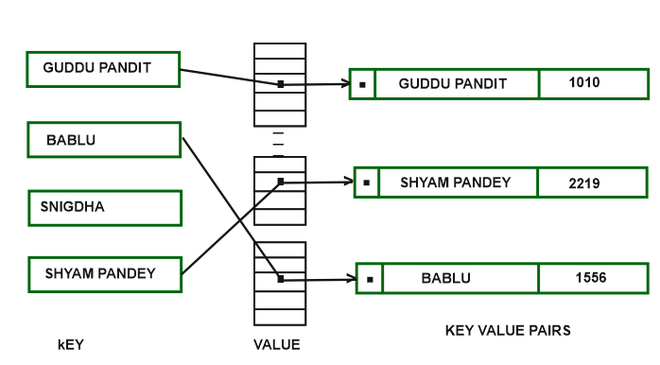Attention reader! Don’t stop learning now. Get hold of all the important Java Foundation and Collections concepts with the Fundamentals of Java and Java Collections Course at a student-friendly price and become industry ready. To complete your preparation from learning a language to DS Algo and many more,  please refer Complete Interview Preparation Course.

Approach:

INSERTION: To insert a key in a hash table, a key will be simply hashed to find the position in which it will be going to insert and after that, the key will be inserted at the head of the list.

DELETION: Just traverse the list with the help of the hash function until the key is found and then simply delete that key from the list

Implementation:

## Java

 `// Java program which implement Hash Tables chaining with``// List Heads`` ` `import` `java.util.Scanner;`` ` `public` `class` `TestHashTables {`` ` `    ``public` `static` `void` `main(String[] args)``    ``{``        ``Scanner sc = ``new` `Scanner(System.in);``        ``System.out.println(``            ``"***** Hash Table Implementation *****"``);``        ``System.out.println(``"Enter size of hash table"``);`` ` `        ``// create object of hash table``        ``HashTablesExample table``            ``= ``new` `HashTablesExample(sc.nextInt());``        ``boolean` `exit = ``false``;`` ` `        ``//  Perform HashTable operations``        ``while` `(!exit) {``            ``System.out.println(``                ``"** Hash Table Operations **"``);``            ``System.out.println(``"1. insert "``);``            ``System.out.println(``"2. remove"``);``            ``System.out.println(``"3. print hash table"``);``            ``System.out.println(``"4. get"``);``            ``System.out.println(``"5. clear"``);``            ``System.out.println(``"6. size"``);`` ` `            ``int` `choice = sc.nextInt();``            ``switch` `(choice) {``            ``case` `1``:``                ``System.out.println(``"Enter key and value"``);``                ``String key = sc.next();``                ``int` `value = sc.nextInt();``                ``table.insert(key, value);``                ``break``;`` ` `            ``case` `2``:``                ``System.out.println(``"Enter key"``);``                ``String key2 = sc.next();``                ``table.remove(key2);``                ``System.out.println(``                    ``"Removed Successfully..."``);``                ``break``;`` ` `            ``case` `3``:``                ``System.out.println(``                    ``"Key-value pairs in the hash table are : "``);``                ``table.printHashTable();``                ``break``;`` ` `            ``case` `4``:``                ``System.out.println(``"Enter key"``);``                ``String key3 = sc.next();``                ``System.out.println(``"Key = "` `+ key3``                                   ``+ ``" Value = "``                                   ``+ table.get(key3));``                ``break``;`` ` `            ``case` `5``:``                ``table.makeEmpty();``                ``System.out.println(``                    ``"Hash Table Cleared Successfully.."``);``                ``break``;`` ` `            ``case` `6``:``                ``System.out.println(``"Size of the table is = "``                                   ``+ table.getSize());``                ``break``;`` ` `            ``default``:``                ``exit = ``true``;``                ``System.out.println(``                    ``"Exited Successfully..."``);``            ``}``        ``}``        ``sc.close();``    ``}``}`` ` `class` `HashTablesExample {``    ``// class linked hash``    ``class` `LinkedHash {``        ``String key;``        ``int` `value;``        ``LinkedHash next;`` ` `        ``// constructor of linked hash``        ``LinkedHash(String key, ``int` `value)``        ``{``            ``this``.key = key;``            ``this``.value = value;``            ``this``.next = ``null``;``        ``}``    ``}`` ` `    ``private` `int` `tableSize;``    ``private` `int` `size;``    ``private` `LinkedHash[] table;`` ` `    ``// constructor of HashTableExample``    ``public` `HashTablesExample(``int` `ts)``    ``{``        ``size = ``0``;``        ``tableSize = ts;``        ``table = ``new` `LinkedHash[tableSize];`` ` `        ``// initializing the hash table with null values``        ``for` `(``int` `i = ``0``; i < tableSize; i++)``            ``table[i] = ``null``;``    ``}`` ` `    ``// method to get the number of key-value pairs in the``    ``// hash table``    ``public` `int` `getSize() { ``return` `size; }`` ` `    ``// method to clear the hash table entry``    ``public` `void` `makeEmpty()``    ``{``        ``for` `(``int` `i = ``0``; i < tableSize; i++)``            ``table[i] = ``null``;``    ``}`` ` `    ``// method to get value of a key``    ``public` `int` `get(String key)``    ``{``        ``int` `value = (myhash(key) % tableSize);``        ``if` `(table[value] == ``null``)``            ``return` `-``1``;``        ``else` `{``            ``LinkedHash current = table[value];``            ``while` `(current != ``null``                   ``&& !current.key.equals(key)) {``                ``current = current.next;``            ``}``            ``if` `(current == ``null``) {``                ``return` `-``1``;``            ``}``            ``else` `{``                ``return` `current.value;``            ``}``        ``}``    ``}`` ` `    ``// method to insert a value in a hash table``    ``public` `void` `insert(String key, ``int` `value)``    ``{``        ``int` `hash = (myhash(key) % tableSize);``        ``if` `(table[hash] == ``null``) {``            ``table[hash] = ``new` `LinkedHash(key, value);``        ``}``        ``else` `{``            ``LinkedHash entry = table[hash];``            ``while` `(entry.next != ``null``                   ``&& !entry.key.equals(key)) {``                ``entry = entry.next;``            ``}``            ``if` `(entry.key.equals(key)) {``                ``entry.value = value;``            ``}``            ``else` `{``                ``entry.next = ``new` `LinkedHash(key, value);``            ``}``        ``}``        ``size++;``    ``}`` ` `    ``// method to remove the value with the specified key``    ``public` `void` `remove(String key)``    ``{``        ``int` `value = (myhash(key) % tableSize);``        ``if` `(table[value] != ``null``) {``            ``LinkedHash prev = ``null``;``            ``LinkedHash current = table[value];``            ``while` `(current.next != ``null``                   ``&& !current.key.equals(key)) {``                ``prev = current;``                ``current = current.next;``            ``}``            ``if` `(current.key.equals(key)) {``                ``if` `(prev == ``null``) {``                    ``table[value] = current.next;``                ``}``                ``else` `{``                    ``prev.next = current.next;``                ``}``                ``size--;``            ``}``        ``}``    ``}`` ` `    ``// method which gives a hash value from a given``    ``// specified string``    ``private` `int` `myhash(String x)``    ``{``        ``int` `value = x.hashCode();``        ``value %= tableSize;``        ``if` `(value < ``0``) {``            ``value = value + tableSize;``        ``}``        ``return` `value;``    ``}`` ` `    ``// method to print the value of hash table``    ``public` `void` `printHashTable()``    ``{``        ``for` `(``int` `i = ``0``; i < tableSize; i++) {``            ``LinkedHash current = table[i];``            ``while` `(current != ``null``) {``                ``System.out.println(``                    ``"Value = "` `+ current.value + ``" "``                    ``+ ``"Key = "` `+ current.key);``                ``current = current.next;``            ``}``        ``}``        ``System.out.println();``    ``}``}`

Output :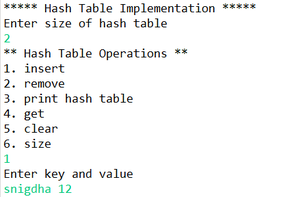insert into hash table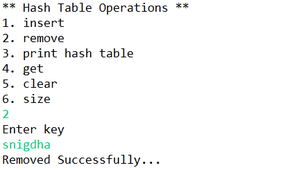remove from hash table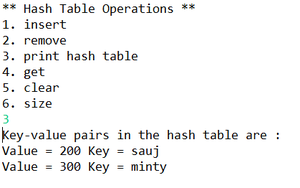print the key-value pair of hash table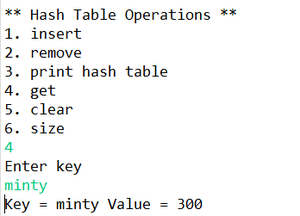get the value from specified key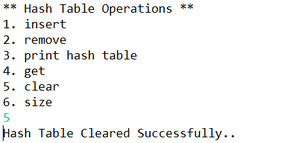clear the hash table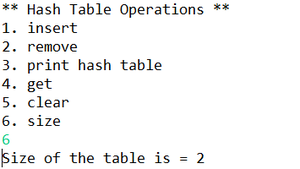size of the hash table

My Personal Notes arrow_drop_up